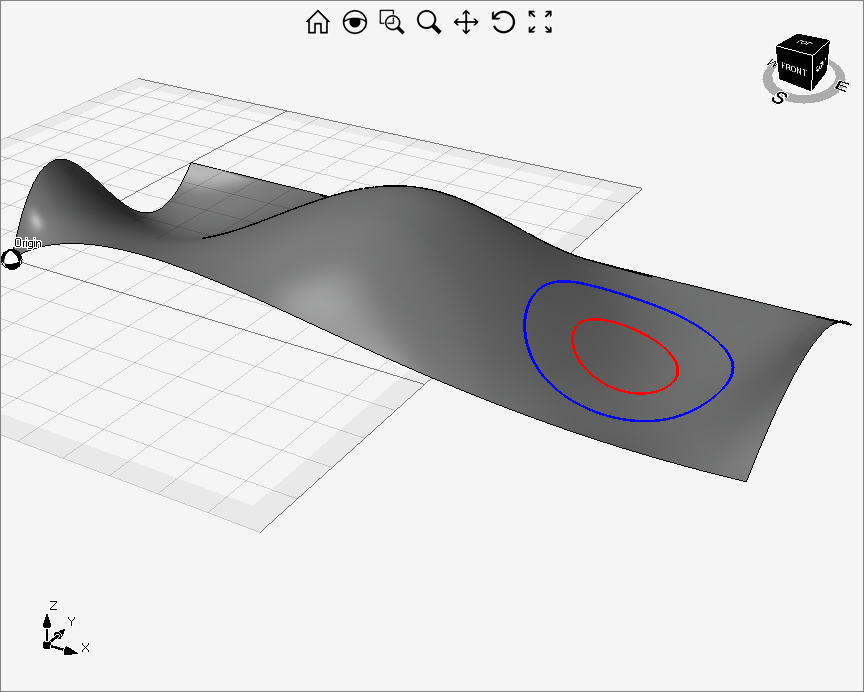# Offsetting a Curve on a Surface

The proposed solution is not natively supported and may not work in all scenarios and versions.

With the sample code reported in this article, you can offset a curve that lies on a non-planar surface.

First of all, it creates a ruled surface normal to the surface in which the curve lies. Then, it offsets the ruled surface and then it finds the offset curve as the intersection between the starting surface and the offsetted ruled surface.

The method proposed in this article makes use of some parameters, such as the height of the ruled surface and the offset tolerance, that are no constant and must be adapted based on the geometry of the specific surface.The code is reported below.

``````//offSet parameters, they may change with the choosen surface and curve
double offSetAmount = 10;
double ruledHeight = 5;
double tolerance = 0.1;

rda.DoWork();
Surface surface = rda.Entities as Surface;
Curve curve = rda.Entities as Curve;
//creates the ruled surface

curve.Regen(0.01);
List<Point3D> curveVertex = curve.Vertices.ToList();
List<Point3D> firstCurveVertex = new List<Point3D>();
List<Point3D> secondCurveVertex = new List<Point3D>();
Vector3D normal;
foreach (Point3D pt in curveVertex)
{
surface.Project(pt, out Point2D proj);
normal = surface.Normal(proj);
normal.Normalize();
}
firstCurveVertex.Add(firstCurveVertex); //comment this line if the curve to offset is open
secondCurveVertex.Add(secondCurveVertex); //comment this line if the curve to offset is open
Curve cv1 = Curve.CubicSplineInterpolation(firstCurveVertex);
Curve cv2 = Curve.CubicSplineInterpolation(secondCurveVertex);
Surface ruled = Surface.Ruled(cv1, cv2);

//offsets the ruled surface

ruled.Offset(offSetAmount, tolerance, out Surface offSetSurface);

//finds the offsetted curve as intersection between the ruled surface and the starting one

Surface.Intersection(new List<Surface> {surface}, new List<Surface> {offSetSurface}, tolerance,out ICurve[] curveOffset);
curve.LineWeight = 3;
curve.LineWeightMethod = colorMethodType.byEntity;
foreach (Entity ent in curveOffset)
{
ent.LineWeight = 3;
ent.LineWeightMethod = colorMethodType.byEntity;
}``````

The ruled surface's normals direction (inward/outward) depend both on the initial curve direction and on the starting surface normal.

If the curve is oriented counterclockwise with respect to the surface's normal then the ruled surface has the normals pointing outward, otherwise, the ruled surface's normals point inward.

To change this default behavior you can call:

`` ruled.ReverseU(); ``

For certain types of curves, creating a Ruled surface may not be the best choice and the offset can fail. A viable alternative you can try to use the Loft() method to create the offset surface instead of the Ruled() one. Here is the code:

``````...
List<ICurve> normalLines = new List<ICurve>();
for (int i = 0; i < firstCurveVertex.Count; i++)
{
}
ruled = Surface.Loft(normalLines);
ruled.Offset(offSetAmount, tolerance, out Surface offSetSurface);
...

``````